## Current Divider Equation:

In a parallel circuit, the Current Division in all branches. Thus, a parallel circuit acts as a current divider. The total current entering into the parallel branches is divided into the branches currents according to the resistance values. The branch having higher resistance allows lesser current, and the branch with lower resistance allows more current. Let us find the Current Divider Equation in the parallel circuit shown in Fig. 1.32.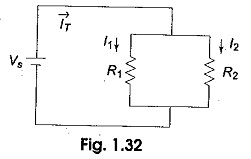The voltage applied across each resistor is Vs. The current passing through each resistor is given by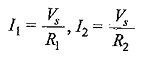If RT is the total resistance, which is given by R1R2/(R1+R2),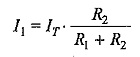From the above equations, we can conclude that the current in any branch is equal to the ratio of the opposite branch resistance to the total resistance value, multiplied by the total current in the circuit. In general, if the circuit consists of m branches, the Current Divider Equation in any branch can be determined by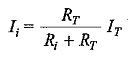where

• Irepresents the current in the ith branch
• Ri is the resistance in the ith branch
• RT is the total parallel resistance to the ith branch and
• Iis the total current entering the circuit.

### Formula of Power in Parallel Circuit:

The total power supplied by the source in any parallel resistive circuit is equal to the sum of the powers in each resistor in parallel, i.e.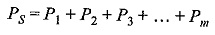where m is the number of resistors in parallel,Ps is the total power and Pm is the power in the last resistor.

Scroll to Top